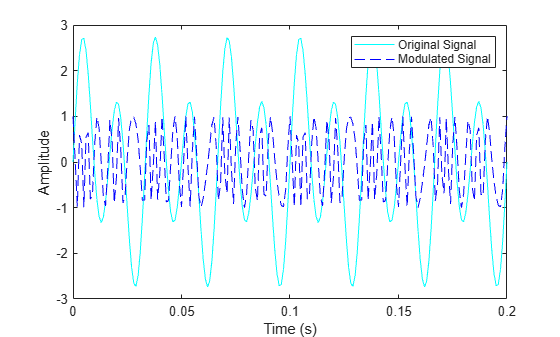Documentation

### This is machine translation

Mouseover text to see original. Click the button below to return to the English version of the page.

# fmmod

Frequency modulation

## Syntax

```y = fmmod(x,Fc,Fs,freqdev) y = fmmod(x,Fc,Fs,freqdev,ini_phase) ```

## Description

`y = fmmod(x,Fc,Fs,freqdev)` modulates the message signal `x` using frequency modulation. The carrier signal has frequency `Fc` (Hz) and sampling rate `Fs` (Hz), where `Fs` must be at least `2*Fc`. The `freqdev` argument is the frequency deviation constant (Hz) of the modulated signal.

`y = fmmod(x,Fc,Fs,freqdev,ini_phase)` specifies the initial phase of the modulated signal, in radians.

## Examples

collapse all

Set the sample rate and carrier frequency. Generate a time vector having a duration of 0.2 s.

```fs = 1000; fc = 200; t = (0:1/fs:0.2)';```

Create a sinusoidal signal.

`x = sin(2*pi*30*t)+2*sin(2*pi*60*t);`

Set the frequency deviation to 50 Hz.

`fDev = 50;`

Frequency modulate `x`.

`y = fmmod(x,fc,fs,fDev);`

Demodulate `z`.

`z = fmdemod(y,fc,fs,fDev); % Demodulate both channels.`

Plot the original and demodulated signals.

```plot(t,x,'c',t,z,'b--'); xlabel('Time (s)') ylabel('Amplitude') legend('Original Signal','Demodulated Signal')```The demodulated signal is well aligned with the original.